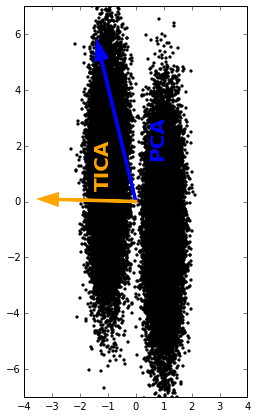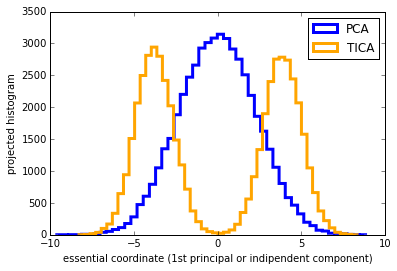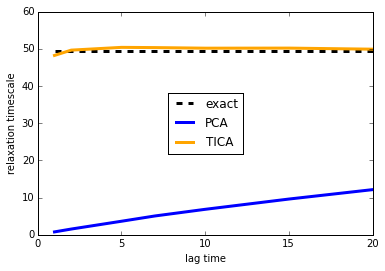# Time-lagged independent component analysis (TICA)¶

Frank Noefrank.noe@fu-berlin.de
FU Berlin, Arnimallee 6, 14195 Berlin, Germany

 Summary: The time-lagged independent component analysis (TICA) defines a linear transform of some (usually high-dimensional) set of input coordinates to some (usually low-dimensional) set of output coordinates. The transform is chosen such that amongst all linear transforms, TICA maximizes the autocorrelation of transformed coordinates. In other words, TICA finds a maximally slow subspace, or a subspace of good reaction coordinates when the input coordinates come from molecular dynamics. TICA is an excellent choice to process high-dimensional input data such as to prepare it for Markov model construction.

TICA was initially introduced in the signal processing literature  - see also  for an extensive review. TICA was introduced into molecular dynamics as a method for extracting slow order parameters independently by  and . It has been shown  that TICA solves a variational approach  by which it finds an optimal approximation to the eigenvalues and eigenfunctions of the Markov operator underlying the molecular dynamics data. Hence, TICA is ideally suited to prepare data for Markov model construction.

## time-lagged independent component analysis¶

We consider a $d$-dimensional vector of input data, called $\mathbf{r}(t)=(r_{i}(t))_{i=1,...,D}$. Here, $t$ is an integer from $\{1...N\}$ denoting the time step. We assume that the data is mean-free, i.e. from a general input vector $\tilde{\mathbf{r}}(t)$, we first obtain: $$\mathbf{r}(t)=\tilde{\mathbf{r}}(t)-\langle\tilde{\mathbf{r}}(t)\rangle_{t} \:\:\:\:(1)$$ where $\langle\tilde{\mathbf{r}}(t)\rangle_{t}$ is the data mean.

We first compute the covariance matrices from the data: $$c_{ij}(\tau)=\langle r_{i}(t)\: r_{j}(t+\tau)\rangle_{t} \:\:\:\:(2)$$ where $\tau$ is the lag time. We will need two matrices for TICA, for the choices $\tau=0$ and another positive value of $\tau$. $\langle\cdot\rangle_{t}$ denotes the time average. We can evaluate it as follows: $$c_{ij}(\tau)=\frac{1}{N-\tau-1}\sum_{t=1}^{N-\tau}r_{i}(t)\, r_{j}(t+\tau). \:\:\:\:(3)$$ It is easy to verify that $\mathbf{C}(0)$ is a symmetric matrix. For algebraic reasons we will need that $\mathbf{C}(\tau)$ is also symmetric, which is not automatically guaranteed when estimating it from a finite data set. Therefore we enforce symmetry from a data-computed matrix $\mathbf{C}_{d}(\tau)$: $$\mathbf{C}(\tau)=\frac{1}{2}\left(\mathbf{C}_{d}(\tau)+\mathbf{C}_{d}^{\top}(\tau)\right). \:\:\:\:(4)$$ Now we solve the generalized eigenvalue problem: $$\mathbf{C}(\tau)\:\mathbf{U}=\mathbf{C}(0)\:\mathbf{U}\:\boldsymbol{\Lambda} \:\:\:\:(5)$$ where $U$ is the eigenvector-matrix containing the independent components (ICs) in the columns and $\Lambda$ is a diagonal eigenvalue matrix. This problem can be solved by an appropriate generalized eigenvalue solver (directly).

Now, the data can be projected onto the TICA space: $$\mathbf{z}^{\top}(t)=\mathbf{r}^{\top}(t)\mathbf{U} \:\:\:\:(6)$$ in this step, we can perform a dimension reduction by selecting only a sub-matrix $\mathbf{U}$ consisting of the first $m$ columns of the full-rank $\mathbf{U}$.

## Amuse algorithm¶

The generalized eigenvalue problem can be solved in two steps. The two step procedure is called AMUSE algorithm  and works as follows:

1. Solve the simple PCA Eigenvalue problem $\mathbf{C}(0)\:\mathbf{W}=\mathbf{W}\:\boldsymbol{\Sigma}$, where $\mathbf{W}$ is the eigenvector matrix with principal components and $\boldsymbol{\Sigma}$ are their variances (diagonal Eigenvalue matrix).

2. Transform the mean-free data $\mathbf{r}(t)$ onto principal components $\mathbf{y}(t)=\mathbf{W}\:\mathbf{r}(t)$.

3. Normalize the principal components: $\mathbf{y}'(t)=\boldsymbol{\Sigma}^{-1}\mathbf{y}(t)$

4. Compute the symmetrized time-lagged covariance matrix of the normalized PCs: $\mathbf{C}_{sym}^{y}(\tau)=\frac{1}{2}\left[\mathbf{C}^{y}(\tau)+(\mathbf{C}^{y}(\tau))^{\top}\right]$

5. Perform an eigenvalue decomposition of $\mathbf{C}_{sym}^{y}(\tau)$ to obtain the eigenvector matrix $\mathbf{V}$ and project the trajectory onto the dominant eigenvectors to obtain $\mathbf{z}(t)$.

In summary, we can write the transformation equation in three linear transforms: $$\mathbf{z}^{\top}(t)=\mathbf{r}^{\top}(t)\mathbf{U}=\mathbf{r}^{\top}(t)\mathbf{W}\boldsymbol{\Sigma}^{-1}\mathbf{V}. \:\:\:\:(7)$$

TICA will be used as a dimension reduction technique. Only the dominant TICA components will be used to go to the next step. In order to reduce the dimension, use only a few columns of $\mathbf{U}$.

#### Citing TICA¶

The TICA method was first introduced in . TICA was introduced into the molecular dynamics literature and as a method for Markov model estimation independently in two papers: . The fact that TICA is an optimal approximation to the Markov operator’s eigenvalues and eigenfunction was shown in .

## Example: TICA versus PCA in a stretched double-well potential¶

In :
import numpy as np
import pyemma.msm as msm
import pyemma.msm.generation as msmgen
import pyemma.msm.analysis as msmana
import pyemma.coordinates as coor
import matplotlib.pylab as plt
%pylab inline

Populating the interactive namespace from numpy and matplotlib

WARNING: pylab import has clobbered these variables: ['plt']
%matplotlib prevents importing * from pylab and numpy

In :
def assign(X, cc):
T = X.shape
I = np.zeros((T),dtype=int)
for t in range(T):
dists = X[t] - cc
dists = dists ** 2
I[t] = np.argmin(dists)
return I


Test data

We generate a Hidden-Markov Model with two Gaussians output functions in two dimensions, in such a way that the maximum variance direction differs from the direction of maximum autocorrelation.

In :
P = np.array([[0.99, 0.01],
[0.01, 0.99]])
T = 50000
means = [np.array([-1,1]), np.array([1,-1])]
widths = [np.array([0.3,2]),np.array([0.3,2])]

In :
# continuous trajectory
X = np.zeros((T, 2))
# hidden trajectory
dtraj = msmgen.generate_traj(P, T)
for t in range(T):
s = dtraj[t]
X[t,0] = widths[s] * numpy.random.randn() + means[s]
X[t,1] = widths[s] * numpy.random.randn() + means[s]


Perform PCA and TICA

We first compute the PCA transform of our trajectory data, and store eigenvalues (variances) and eigenvectors (principal components):

In :
pca = coor.pca(data = X)
pc = pca.eigenvectors
S = pca.eigenvalues

2015-04-16 22:21:46,367 PCA[0x108df29d0] INFO     Running PCA on 2 dimensional input

In :
km = coor.cluster_kmeans(data = X, k=100)

2015-04-16 22:21:46,385 KmeansClustering[0x108df28d0] WARNING  K-means implementation is currently memory inefficient. This calculation needs 1 megabytes of main memory. If you get a memory error, try using a larger stride.
2015-04-16 22:21:46,387 KmeansClustering[0x108df28d0] INFO     Accumulated all data, running kmeans on (50000, 2)


Next, we compute the TICA transform and store eigenvalues (autocorrelations) and eigenvectors (independent components)

In :
tica = coor.tica(data = X)
ic = tica.eigenvectors
L = tica.eigenvalues

2015-04-16 22:21:55,385 TICA[0x108df2ed0] INFO     Running TICA with tau=10; Estimating two covariance matrices with dimension (2, 2)
2015-04-16 22:21:55,388 TICA[0x108df2ed0] INFO     calculated mean.
2015-04-16 22:21:55,391 TICA[0x108df2ed0] INFO     finished calculation of Cov and Cov_tau.
2015-04-16 22:21:55,391 TICA[0x108df2ed0] INFO     diagonalize Cov and Cov_tau
2015-04-16 22:21:55,394 TICA[0x108df2ed0] INFO     finished diagonalisation.


Now, we plot our results. The data is shown in the form of a scatter plot, and the principal components / independent components are shown as arrows, scaled by the magnitude of the associated eigenvalue

In :
def draw_arrow(a, v, color):
plt.arrow(0, 0, a*v, a*v, color=color, width=0.02, linewidth=3)

In :
plt.figure(figsize=(4,7))
scatter(X[:,0], X[:,1], marker = '.', color='black')
draw_arrow(S, pc[:,0], color='blue')
#draw_arrow(S, pc[:,1], color='blue')
plt.text(0.5, 2.5, 'PCA', color='blue', fontsize = 20, fontweight='bold', rotation='vertical')
draw_arrow(4*L, ic[:,0], color='orange')
#draw_arrow(4*L, ic[:,1], color='orange')
plt.text(-1.5, 1.7, 'TICA', color='orange', fontsize = 20, fontweight='bold', rotation='vertical')
xlim(-4,4)
ylim(-7,7)

Out:
(-7, 7)We can clearly see that the first PCA component is along the direct of maximal variance in the data, which is in this case not very informative for resolving the rare event that takes place between the two elongated Normal distributions. The second principal components is orthogonal to the first component in the real space.

TICA successfully identifies the slow reaction coordinate. The corresponding eigenvalue (autocorrelation) is nearly one as the associated process is slow. The second TICA component is orthogonal in a scaled space and thus appears nonorthogonal in the real space. The eigenvalue associated with the second TICA component is nearly zero, indicating that no second slow process exists

Projection

Now we project the input data onto the first principal or independent component, respectively:

In :
Ypca = pca.get_output()[:,0]
Ytica = tica.get_output()[:,0]


For better visual clarity, the TICA trajectory is just scaled by a multiple. We can see that the TICA projection clearly distinguishes between the two metastable states, while the PCA projection lumps them together:

In :
hist(Ypca, bins=50, histtype='step', linewidth=3, label='PCA', color='blue')
hist(4*Ytica, bins=50, histtype='step', linewidth=3, label='TICA', color='orange')
xlabel('essential coordinate (1st principal or indipendent component)')
ylabel('projected histogram')
legend()

Out:
<matplotlib.legend.Legend at 0x10bcd0f10>1D-MSM

The advantage of a projection that preserves the slow process directions is immediately seen when building a Markov state model along the first coordinate, either for the PCA or TICA projection:

In :
cc_pca = np.linspace(np.min(Ypca), np.max(Ypca))
I_pca = assign(Ypca[:,None], cc_pca)
cc_tica = np.linspace(np.min(Ypca), np.max(Ypca))
I_tica = assign(Ytica[:,None], cc_tica)

In :
lags = [1,2,5,7,10,15,20]
its_pca = msm.its([I_pca], lags)
its_tica = msm.its([I_tica], lags)
plot([1,20],[msmana.timescales(P),msmana.timescales(P)], linewidth=3, linestyle='dashed', color='black', label='exact')
plot(its_pca.get_lagtimes(), its_pca.get_timescales()[:,0], linewidth=3, color='blue', label='PCA')
plot(its_tica.get_lagtimes(), its_tica.get_timescales()[:,0], linewidth=3, color='orange', label='TICA')
xlabel('lag time')
ylabel('relaxation timescale')
legend(loc='center')

/Users/noe/data/software_projects/pyemma/pyemma/msm/ui/timescales.py:207: DeprecationWarning: get_lagtimes() is deprecated. Use lagtimes
warnings.warn('get_lagtimes() is deprecated. Use lagtimes', DeprecationWarning)
/Users/noe/data/software_projects/pyemma/pyemma/msm/ui/timescales.py:207: DeprecationWarning: get_lagtimes() is deprecated. Use lagtimes
warnings.warn('get_lagtimes() is deprecated. Use lagtimes', DeprecationWarning)

Out:
<matplotlib.legend.Legend at 0x10c0f0550>While the 1D-Markov model of the PCA projection gives a very poor and slowly converging estimate of the relaxation timescales, the 1D-Markov model of the TICA projection recovers the true timescale accurately and for short lag times.

In [ ]:



## Bibliography¶

: Aapo Hyvärinen, Juha Karhunen, Erkki Oja: Independent Component Analysis. John Wiley \& Sons. (2001).

: Molgedey, L. and Schuster, H. G.: Separation of a mixture of independent signals using time delayed correlations. Phys. Rev. Lett. 72, 3634-3637 (1994).

: F. Noé and F. Nüske: A variational approach to modeling slow processes in stochastic dynamical systems. SIAM Multiscale Model. Simul. 11, 635-655 (2013).

: F. Nüske, B. Keller, G. Pérez-Hernández, A. S. J. S. Mey and F. Noé: Variational Approach to Molecular Kinetics. J. Chem. Theory Comput. 10, 1739-1752 (2014).

: G. Perez-Hernandez, F. Paul, T. Giorgino, G. {de Fabritiis} and Frank Noé: Identification of slow molecular order parameters for Markov model construction. J. Chem. Phys. 139, 015102 (2013).

: C. R. Schwantes and V. S. Pande: Improvements in Markov State Model Construction Reveal Many Non-Native Interactions in the Folding of NTL9. J. Chem. Theory Comput. 9, 2000-2009 (2013).